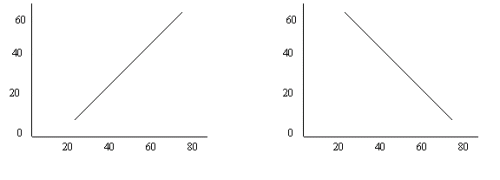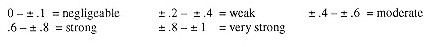# Determining Correlation

A correlation is used to estimate the relationship between two characteristics. If we are dealing with two ordinal or one ordinal and one numerical (interval or ratio) characteristic, then the correct correlation to use is the Spearman rank correlation, named after the statistician, also known as rho or rs. Computer software programs such as SPSS will execute the tedious task of calculating Spearman’s rho very easily.

Correlations range from –1 to +1. At these extremes, the correlation between the two characteristics is perfect, although it is negative or inverse in the first instance. A perfect correlation is one where the two characteristics increase or decrease by the same amount. This is a linear relationship as illustrated below.A correlation coefficient of 0 therefore refers to a situation where no relationship exists between the two characteristics. In other words, changes in one characteristic cannot be explained by the changes occurring in the second characteristic. A common way of interpreting the strength of a correlation is as follows:However, in most tourism and hospitality related research, corrections between ± .26 to ± .5 are generally considered to be quite high, with strong or very strong correlations rarely found.

The variation in one characteristic can be predicted if we know the value of correlation, since we can calculate the coefficient of determination or r2.

If we have a correlation of  ±.5, then  ±.52 = .25. We can therefore conclude that 25% of the variation in one characteristics can be predicted by the value of the second measure.

Let us take a look at an example to make these interpretations clearer. Let us assume we wish to determine whether pleasure travellers who are interested in staying in first class hotels are motivated because they wish to indulge in luxury or because of some other motivation, such as the identification of luxury hotels with big modern cities. By running a Spearman correlation on these three ordinal variables that asked respondents to rate the importance of each, we obtain the following output:

 First class hotel Big modern cities Indulging in luxury Spearman's rho First class hotel Correlation Coefficient 1.000 .229** .615** Sig. (2-tailed) . .000 .000 N 1472 1467 1468 Big modern cities Correlation Coefficient .229** 1.000 .246** Sig. (2-tailed) .000 . .000 N 1467 1480 1476 Indulging in luxury Correlation Coefficient .615** .246** 1.000 Sig. (2-tailed) .000 .000 . N 1468 1476 1487

**Correlation is significant at the .01 level (2-tailed).

If we look at the first pair of variables (‘big modern cities’ by ‘first class hotel’) we note that the correlation coefficient is .229 or ‘weak’, while the statistical significance is very high (.000 is so small that the number itself is cut off) Indeed, as the footnote says, the results are significant at the .01 level. This can also be interpreted as "the level of confidence is 99%"(1 - .01 = .99). Finally, the output informs us that 1472 of our survey respondents answered both questions.

Similarly, the second pair of variables (‘indulging in luxury’ by ‘first class hotel’) show us a strong correlation (indeed, unusually high for this type of research at .615) that is statistically significant and where 1468 respondents answered both questions.

We can therefore conclude that ‘indulging in luxury’ is strongly correlated with the choice of first class hotel accommodation and that 38% (r2 = .615 x .615) of the variance in first class hotels is determined by this factor. Furthermore, this finding is statistically significant, which means we can reject the null hypothesis that there is no relationship between the importance attributed to staying in first class hotels and indulging in luxury. We can further conclude that while ‘big modern cities’ are associated with luxury hotels, that correlation is weak with only 5% (r2 = .229 x .229) of the variance in luxury hotels explained by it.